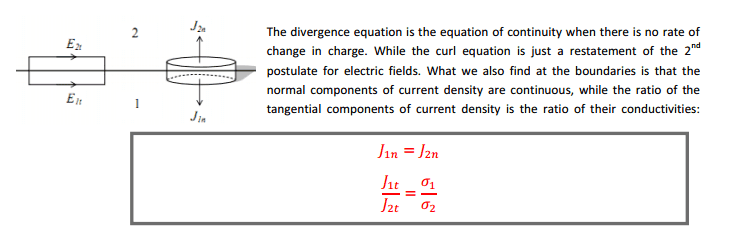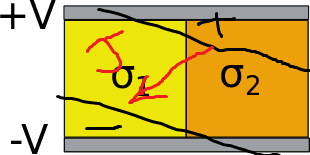# Electromagnetics:boundary condition for steady current density

Although I understand the derivation of boundary condition in case of steady electric current but I did not understand, that the electric field which is in direction of J current density which is generated from permanent potential to have a current in a conductors that is applied between two conducting medium so that the direction of the electric field should be the same as the potential applied is the same on both conductors and the value of J change due to different value of conductivity bet medium while the direction should still the same.

so i did not under stand how electric field and current density change direction although the source that applied on two conductors is the same ?my question what if the field like i draw why the direction of current change although the same source should make the current,electric field in both conductors in the same directionLast edited:

## 1. What is the purpose of boundary conditions in electromagnetics?

Boundary conditions in electromagnetics are used to define the behavior of electromagnetic fields at the interface between different materials or regions. They help to determine how the fields will behave and interact with each other at these boundaries.

## 2. What is the boundary condition for steady current density?

The boundary condition for steady current density states that the total current flowing into a given volume must be equal to the total current flowing out of that volume. This ensures that charge is conserved and that the current density remains constant over time.

## 3. How is the boundary condition for steady current density applied in practice?

In practice, the boundary condition for steady current density is applied by using mathematical equations to calculate the values of the electric and magnetic fields at the boundary between two materials or regions. These equations take into account the properties of the materials and the geometry of the boundary.

## 4. What happens if the boundary condition for steady current density is not satisfied?

If the boundary condition for steady current density is not satisfied, it means that there is a violation of charge conservation and the current density is not constant. This can lead to incorrect calculations and predictions of electromagnetic behavior in the system.

## 5. Are there any exceptions to the boundary condition for steady current density?

Yes, there are some cases where the boundary condition for steady current density may not apply. For example, in the presence of time-varying fields or in highly conductive materials, the steady current density assumption may not be valid and other boundary conditions may need to be used.